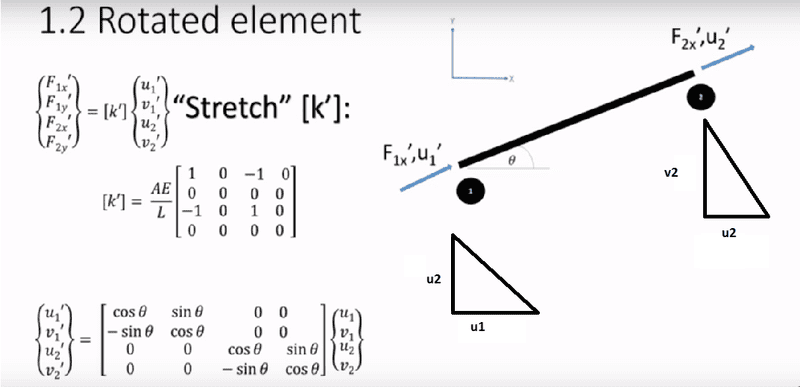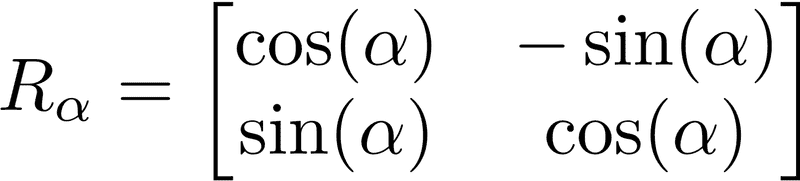# Matrix of rotated elements (stiffness matrix)

Good day All
I have a doubt regarding the derivation of the following matrixaccording to my basic understanding we want to go from the basis u1 v1 u2 v2 to the basis u'1 v'1 u'2 v'2, and for doing so we use the rotation matrix

the rotation matrix is the following and the angle theta is positivebut i still can 't understand why the signs are inverted?
any help would be highly appreciated

#### Attachments

•